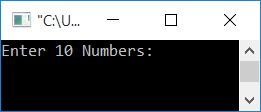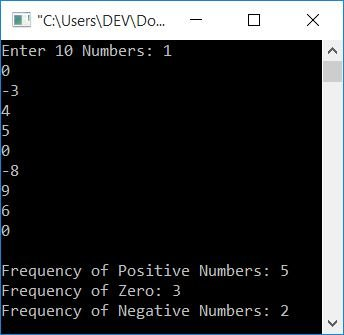# C++ Program to Count Positive, Zero, and Negative Numbers

In this article, you will learn and get code on counting positive, zero, and negative numbers in C++. The program is created in the following ways:

## Count positive, negative, and zero in C++

The program given below counts the occurrence (frequency) of positive, zero, and negative numbers from the given set of numbers entered by the user (at run-time) in C++ programming.

The question is: write a program in C++ that counts and prints the frequencies of positive, zero, and negative numbers. The answer to this question is given below:

```#include<iostream>
using namespace std;
int main()
{
intpos=0, neg=0, zer=0, i, arr;
cout<<"Enter 10 Numbers: ";
for(i=0; i<10; i++)
cin>>arr[i];
for(i=0; i<10; i++)
{
if(arr[i]>0)
pos++;
else if(arr[i]==0)
zer++;
else
neg++;
}
cout<<"\nFrequency of Positive Numbers: "<<pos;
cout<<"\nFrequency of Zero: "<<zer;
cout<<"\nFrequency of Negative Numbers: "<<neg;
cout<<endl;
return 0;
}```

This program was built and runs under Code::Blocks. Here is its sample run:Now supply any 10 numbers, say 1, 0, -3, 4, 5, 0, -8, 9, 6, 0, and press the ENTER key to see the occurrence or frequency of positive, zero, and negative numbers as shown in the output given below:In the above program, when the user enters 10 numbers (for example: 1, 0, -3, 4, 5, 0, -8, 9, 6, 0), then all the 10 numbers get initialized to arr[] in the following way:

• arr=1
• arr=0
• arr=-3
• arr=4
• and so on, upto
• arr=0

Now the dry run of the above program with these 10 values goes like this:

• Initial values: pos=0, neg=0, zer=0
• Because the first for loop gets executed and 10 numbers gets received the by user.
• So now it is time to execute the second for loop.
• Its initialization part executes at first and only once. So i=0
• Now the condition i<10 or 0<10 evaluates to be true; therefore, program flow goes inside the loop.
• The condition of if, that is, arr[i]>0 or arr>0 or 1>0, evaluates to be true, therefore program flow goes inside if's body and the value of pos gets incremented. Because pos was previously 0, it now equals 1.
• Because the if condition is true, the else if and else block will not be executed.
• So program flow goes back, increments the value of i (now i = 1), and evaluates the condition of the for loop again.
• That is, the condition i<10 or 1<10 again evaluates to be true, therefore, program flow again goes inside the loop.
• and evaluates the condition of if, that is, the condition arr[i]>0 or arr>0 or 0>0 evaluates to be false, therefore program flow evaluates the condition of else if, that is, the condition arr[i]==0 or arr==0 or 0==0 evaluates to be true, therefore program flow goes to else if's body and the value of zer gets incremented. Because zer previously had the value 0, it now has the value 1.
• Now again, the program flow goes back, increments the value of i (now i = 2) and evaluates the condition of the for loop again.
• This process is repeated until the for loop's condition evaluates to false.
• On continuing the dry run of this for loop, here are the values we will get after each evaluation:
• pos=1
• zer=1
• neg=1
• pos=2
• pos=3
• zer=2
• neg=2
• pos=4
• pos=5
• zer=3
• After exiting from the loop, print the value of each variable corresponding to positive, zero, and negative numbers.
• That is, the variable, pos (which corresponds to a positive number's occurrence) holds its value as 5. So there are a total of 5 positive numbers available on the list.
• Similarly, there are a total of 3 zeros and 2 negative numbers in the list.

### Allow the user to define the size of the array

This program allows the user to define the size of the array. That is, how many numbers he/she wants to enter, and then find and print the occurrence of positive, zero, and negative numbers.

```#include<iostream>
using namespace std;
int main()
{
intpos=0, neg=0, zer=0, i, arr, tot;
cout<<"Enter the Size (max. 100): ";
cin>>tot;
cout<<"Enter "<<tot<<" Numbers: ";
for(i=0; i<tot; i++)
{
cin>>arr[i];
if(arr[i]>0)
pos++;
else if(arr[i]==0)
zer++;
else
neg++;
}
cout<<"\nFrequency of Positive Numbers: "<<pos;
cout<<"\nFrequency of Zero: "<<zer;
cout<<"\nFrequency of Negative Numbers: "<<neg;
cout<<endl;
return 0;
}```

Below is its sample run with user input, 5 as size, and 1, -2, 3, 0, 4 as 5 numbers:Note: At the time of receiving numbers, the above program counts positive, zero, and negative numbers. That is, the preceding program handles both receiving and counting with a single for loop.

### Count positive, negative, and zero in C++ using the while loop

This program uses a while loop to receive the input (a list of numbers) and counts the occurrence of positive, zero, and negative numbers.

```#include<iostream>
using namespace std;
int main()
{
intpos=0, neg=0, zer=0, i=0, arr, tot;
cout<<"Enter the Size (max. 100): ";
cin>>tot;
cout<<"Enter "<<tot<<" Numbers: ";
while(i<tot)
{
cin>>arr[i];
if(arr[i]>0)
pos++;
else if(arr[i]==0)
zer++;
else
neg++;
i++;
}
cout<<"\nFrequency of Positive Numbers: "<<pos;
cout<<"\nFrequency of Zero: "<<zer;
cout<<"\nFrequency of Negative Numbers: "<<neg;
cout<<endl;
return 0;
}```

This program produces the same output as the previous program.

#### The same program in different languages

C++ Quiz

« Previous Program Next Program »